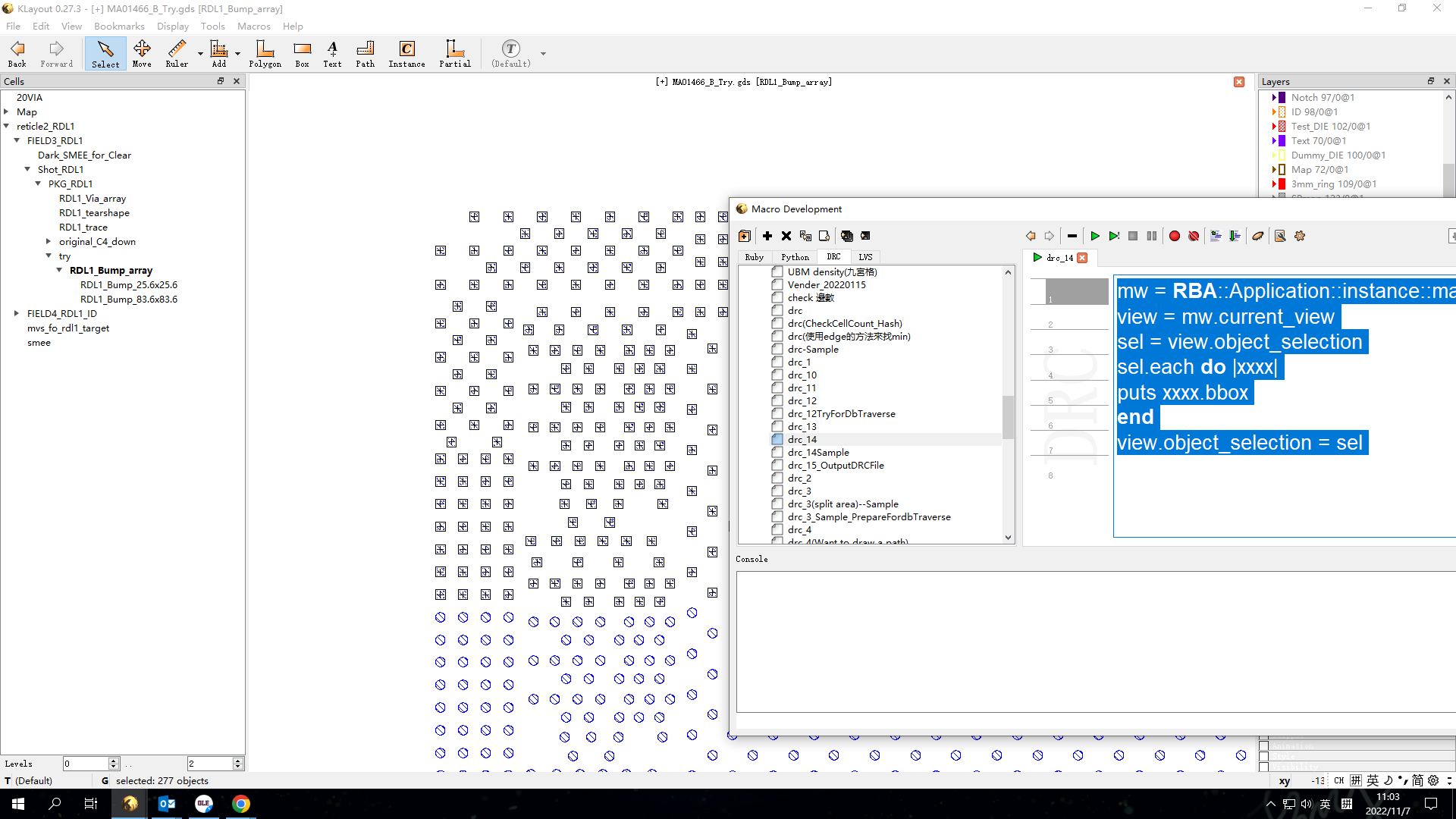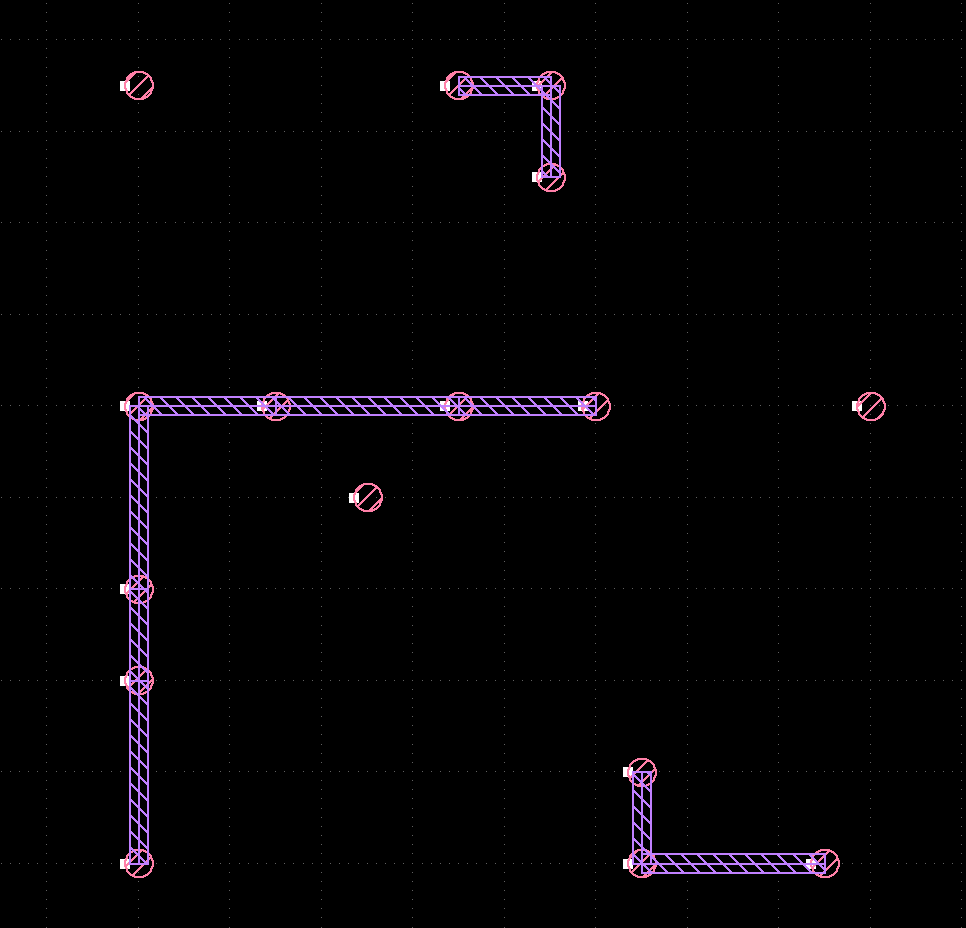# How to export the instance point for the cell been selection?

Hi Sir,
in my case , over 50000 cells into current view , so I just want to get the bbox(or instance point) for some of cell that been user selected ,
may I know how to do that in Ruby DRC?
as below code , I can't get the result...
if any command or nows , please let me know.
Thanks very much.

``````mw = RBA::Application::instance::main_window
view = mw.current_view
sel = view.object_selection
sel.each do |xxxx|
puts xxxx.bbox
end
view.object_selection = sel
``````• I'm thinking that you want to query the instance property list for origin coords?

How, no idea.
• I think so too ... but why DRC?

• Hi Matthias,
I have no idea/powerful for fully Ruby coding.
so , I always using DRC +Ruby to do what I need.
in this case , I want to make line /path connect between some part of bump which is I selected.
as below , the code can help me to do the daisy chain loop .
But , that is not very smart ,I still need to connect some of bump pad , one by one.
That is reason I want to export some of instance point (position of cell origin)
and code the result to make Dpath.
Maybe that is not good way , but that is what the way I can do ....:P

``````layout_view = RBA::Application.instance.main_window.current_view
cell_view = layout_view.active_cellview
layout = cell_view.layout
viewed_cell = cell_view.cell
layer = input(70,0)
width=15
linegap=600
layer = layout.layer(73,0)
point=Array.new()
point << "#{xx.bbox.center.y / 1000.0},#{ xx.bbox.center.x / 1000.0}"
end
point=point.sort.uniq
totally=point.size
for i in 0..(totally-2)
point1x=point[i].split(",").to_f
point1y=point[i].split(",").to_f
point2x=point[i+1].split(",").to_f
point2y=point[i+1].split(",").to_f
if ((point1x==point2x || point1y==point2y)&&(([point1x,point2x].max- [point1x,point2x].min) <linegap)) || (((point1x-point2x)**2 +(point1y-point2y)**2)**0.5)<linegap then
point1 = RBA::DPoint.new(point1x,point1y)
point2 = RBA::DPoint.new(point2x,point2y)
viewed_cell.shapes(layer).insert(RBA::DPath.new([point1, point2],width))
end
end
``````
• edited November 2022

Hello JW,

This topic interests me. The code insure the connection between 2 bumps if the distance under 600.
I imagine, maybe manually sorting can be skipped if the jugde condition be more solid. It will be fun if you
can share the criteria for bumps to be connected or not.

• Hi @Vincent Lin
In other code , I using all the bump coordinate and do-loop to finished almost chain loop.(bump count >10000)
after the code (process all the bump) I still need to manual process chain group around ~200.
Cause I have process almost bump cell , it is why I using this odd flow ---manually chooses the bumps which not been process in first step and filter some connnect base on the bump distance by 600um.

• @jiunnweiyeh

I'm a bit confused because initially you meant selected instances, but from the DRC script you do something with "interact" and work on shapes ...

Anyway, here is a script that lets you select instances and connects their centers if below a certain threshold. Note that this is not a DRC script but a Ruby macro. It provides undo/redo support and you can configure it to show up as a menu item:

``````# NOTE: this is a Ruby script, not DRC!

# the distance of cell centers below which to create a wire
distance = 600.0

# the width of the wire
wire_width = 40.0

view = RBA::LayoutView::current
cv = view.active_cellview_index
ly = view.cellview(cv).layout

# place results on layer 2/0 in this case

output_layer = ly.layer(2, 0)
output = ly.top_cell.shapes(output_layer)

# fetch the center positions of all selected instances

positions = []

view.each_object_selected do |sel|
if sel.is_cell_inst?
positions << (sel.dtrans * sel.inst.dbbox).center
end
end

if positions.size >= 2

begin

# provide undo/redo support

# sort by x first

def xfirst(a,b)
return a.y != b.y ? a.y <=> b.y : a.x <=> b.x
end

sorted = positions.sort { |a,b| xfirst(a, b) }
(sorted.size - 1).times do |i|
p1 = sorted[i]
p2 = sorted[i + 1]
if p1.y == p2.y && (p1.x - p2.x).abs < distance
output.insert(RBA::DPath::new([p1, p2], wire_width))
end
end

# sort by y first

def yfirst(a,b)
return a.x != b.x ? a.x <=> b.x : a.y <=> b.y
end

sorted = positions.sort { |a,b| yfirst(a, b) }
(sorted.size - 1).times do |i|
p1 = sorted[i]
p2 = sorted[i + 1]
if p1.x == p2.x && (p1.y - p2.y).abs < distance
output.insert(RBA::DPath::new([p1, p2], wire_width))
end
end

ensure
view.commit
end

end
``````

This script gives me this in one example of mine:Matthias

• @Matthias
Thanks a lot , It is workabe.